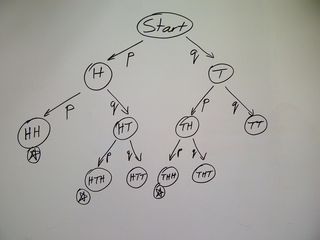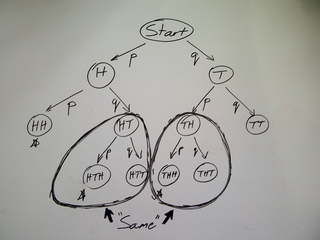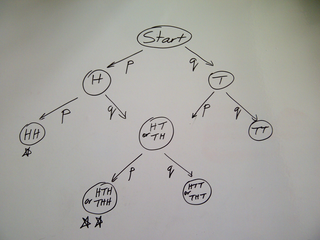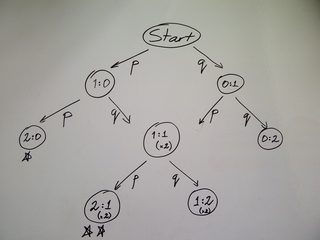# Decision Trees In GamesSubscribe!My lastest posts can be found here:
Previous blog posts:

## Decision Trees in Games (Part 1) - 2011/05/15

A fairly standard exercise in probability is to ask who, under a given scoring system, will win a game given the probability of each move. For example, suppose we toss a coin, and I get a point for every head, and you get a point for every tail. Winner is first to 2.

It's easy if the coin is fair, because the game is symmetrical. It's easy if it's a two headed coin, or a two tailed coin, because then the winner is certain.

But if the coin shows head with probability p (and tail with probability q=1-p) then it's harder. We need the probability of 2 heads before 2 tails.

We can solve this by drawing a tree of what can happen. We start at the top, and draw two arrows, one representing a tail, the other for a head. From each of those places we again draw two arrows, and so on. We stop when we've got two heads, or two tails. The diagram at right shows the result.Decision tree for "First to 2 heads"

We now have all the possibilities, and we can see that there are three ways "H" can win: "HH", "HTH", "THH". By tracing their respective paths down the tree we can see the probability of each combination, and so we get our answer of pp+pqp+qpp, which simplifies to p2+2p2q or p2(1+2q).

We can check this in three particular cases. If the coin is fair (so p=1/2 and q=1/2) then it should evaluate to 1/2. If it's a double headed coin (so p=1 and q=0) then it should evaluate to 1, and if p=0 (so q=1) then it should evaluate to 0.

And it does. That's nice.

This is fine for such a small problem, but it becomes overly cumbersome if we wanted, say, first to 5, instead of just first to 2. But then we realise: when the score is 1:1 we don't care how we got there (although we do care how many ways there were to get there). After "HT" the score is 1:1, and after "TH" the score is again 1:1, and so after that the possible options are all the same. We can collapse the two states "HT" and "TH" into one state, and just remember that there were two ways to get there.

This is reflected in our answer. We have p2 for the straight line to the solution, but there are two ways of going through 1:1, hence 2 lots of p2q.Merging identical sub-trees

For larger cases there are ways to do this algebraically and without the diagrams, but personally, I find that understanding and intuition comes from the diagrams, and the counting arguments are guided by the structure in the diagram, whether I draw the diagram, or simply think of how to draw it.

So in larger cases we can collapse the tree into a more compact structure, which in this case is a directed acyclic graph (DAG) - "directed" because we have arrows showing how we travel from score to score, and "acyclic" because we never return to a previous score.

In a later post we'll look at non-cyclic structures, and then find a surprise in an apparently well-understood game.Decision DAG for "First to 2 heads"

 <<<< Prev <<<< A Matter Of Convention : >>>> Next >>>> Decision Tree For Tennis

 You should follow me on twitter# ContentsSuggest a change ( <-- What does this mean?) / Send me email